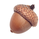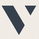# A Useful MA Weighting Function For Controlling Lag & Smoothness25809 views
So far the most widely used moving average with an adjustable weighting function is the Arnaud Legoux moving average ( ALMA ), who uses a Gaussian function as weighting function. Adjustable weighting functions are useful since they allow us to control characteristics of the moving average such as lag and smoothness.

The following moving average has a simple adjustable weighting function that allows the user to have control over the lag and smoothness of the moving average, we will see that it can also be used to get both an SMA and WMA .

A high-resolution gradient is also used to color the moving average, makes it fun to watch, the plot transition between 200 colors, would be tedious to make but everything was made possible using a custom R script, I only needed to copy and paste the R console output in the Pine editor.

Settings
• length : Period of the moving average
• -Lag : Setting decreasing the lag of the moving average
• +Lag : Setting increasing the lag of the moving average

Estimating Existing Moving Averages

The weighting function of this moving average is derived from the calculation of the beta distribution, advantages of such distribution is that unlike a lot of PDF , the beta distribution is defined within a specific range of values (0,1). Parameters alpha and beta controls the shape of the distribution, with alpha introducing negative skewness and beta introducing positive skewness, while higher values of alpha and beta increase kurtosis .

Here -Lag is directly associated to beta while +Lag is associated with alpha. When alpha = beta = 1 the distribution is uniform, and as such can be used to compute a simple moving average .

Moving average with -Lag = +Lag = 1, its impulse response is shown below.

It is also possible to get a WMA by increasing -Lag, thus having -Lag = 2 and +Lag = 1.

Using values of -Lag and +Lag equal to each other allows us to get a symmetrical impulse response, increasing these two values controls the heaviness of the tails of the impulse response.

Here -Lag = +Lag = 3, note that when the impulse response of a moving average is symmetrical its lag is equal to (length-1)/2.

As for the gradient, the color is determined by the value of an RSI using the moving average as input.

I don't promise anything but I will try to respond to your comments
Open-source script

In true TradingView spirit, the author of this script has published it open-source, so traders can understand and verify it. Cheers to the author! You may use it for free, but reuse of this code in a publication is governed by House Rules. You can favorite it to use it on a chart.

Want to use this script on a chart?
Patreon: https://www.patreon.com/alexgrover

You can also check out some of the indicators I made for luxalgo : https://www.tradingview.com/u/LuxAlgo/#published-scriptsThis publication is now featured in our Editors' Picks. In the name of all TradingView traders, thank you for your valuable contribution to the TradingView community, and congrats!